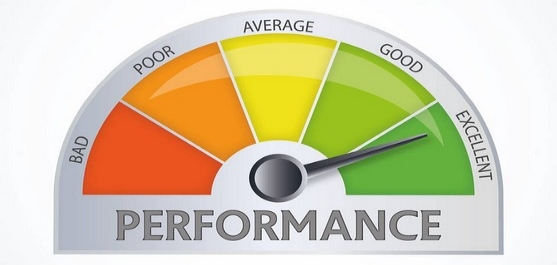## Kaggle evaluation metrics used for regression problems

Posted on Sat 16 February 2019 in Posts • Tagged with machine learning, evaluation, metrics, performance • 7 min readThis post describe evaluation metrics used in Kaggle competitions where problem to solve is has regression nature. Eight different metrics are described, namely: Absolute Error (AE), Mean Absolute Error (MAE), Weighted Mean Absolute Error (WMAE), Pearson Correlation Coefficient, Spearman’s Rank Correlation, Root Mean Squared Error (RMSE), Root Mean Squared Logarithmic Error (RMSLE), Mean Columnwise Root Mean Squared Error (MCRMSE).